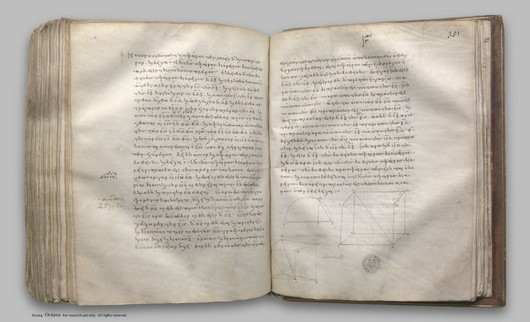## Euclid's Elements, Book XIII

 elem.13.1 If a straight line be cut in extreme and mean ratio, the square on the greater segment added to the half of the whole is five times the square on the half. f. 338 digilib elem.13.2 If the square on a straight line be five times the square on a segment of it, then, when the double of the said segment is cut in extreme and mean ratio, the greater segment is the remaining part of the original straight line. f. 339 digilib elem.13.3 If a straight line be cut in extreme and mean ratio, the square on the lesser segment added to the half of the greater segment is five times the square on the half of the greater segment. f. 340 digilib elem.13.4 If a straight line be cut in extreme and mean ratio, the square on the whole and the square on the lesser segment together are triple of the square on the greater segment. f. 340 digilib elem.13.5 If a straight line be cut in extreme and mean ratio, and there be added to it a straight line equal to the greater segment, the whole straight line has been cut in extreme and mean ratio, and the original straight line is the greater segment. f. 341 digilib elem.13.6 If a rational straight line be cut in extreme and mean ratio, each of the segments is the irrational straight line called apotome. f. 342 digilib elem.13.7 If three angles of an equilateral pentagon, taken either in order or not in order, be equal, the pentagon will be equiangular. f. 345 digilib elem.13.8 If in an equilateral and equiangular pentagon straight lines subtend two angles taken in order, they cut one another in extreme and mean ratio, and their greater segments are equal to the side of the pentagon. f. 346 digilib elem.13.9 If the side of the hexagon and that of the decagon inscribed in the same circle be added together, the whole straight line has been cut in extreme and mean ratio, and its greater segment is the side of the hexagon. f. 347 digilib elem.13.10 If an equilateral pentagon be inscribed in a circle, the square on the side of the pentagon is equal to the squares on the side of the hexagon and on that of the decagon inscribed in the same circle. f. 348 digilib elem.13.11 If in a circle which has its diameter rational an equilateral pentagon be inscribed, the side of the pentagon is the irrational straight line called minor. f. 349 digilib elem.13.12 If an equilateral triangle be inscribed in a circle, the square on the side of the triangle is triple of the square on the radius of the circle. f. 351 digilib elem.13.13 To construct a pyramid, to comprehend it in a given sphere, and to prove that the square on the diameter of the sphere is one and a half times the square on the side of the pyramid. f. 351 digilib elem.13.14 To construct an octahedron and comprehend it in a sphere, as in the preceding case; and to prove that the square on the diameter of the sphere is double of the square on the side of the octahedron. f. 353 digilib elem.13.15 To construct a cube and comprehend it in a sphere, like the pyramid; and to prove that the square on the diameter of the sphere is triple of the square on the side of the cube. f. 355 digilib elem.13.16 To construct an icosahedron and comprehend it in a sphere, like the aforesaid figures; and to prove that the side of the icosahedron is the irrational straight line called minor. f. 356 digilib elem.13.17 To construct a dodecahedron and comprehend it in a sphere, like the aforesaid figures, and to prove that the side of the dodecahedron is the irrational straight line called apotome. f. 358 digilib elem.13.18 To set out the sides of the five figures and to compare them with one another. f. 361 digilib

Clay Mathematics Institute Historical Archive

Published May 8, 2008. Copyright 2008, Clay Mathematics InstituteProposition 13.15WWW claymath.org

You may also enter Greek text in the search box, e.g. cut and paste from the Greek text on this site.